# 3.1: Required Math Concepts

$$\newcommand{\vecs}{\overset { \rightharpoonup} {\mathbf{#1}} }$$ $$\newcommand{\vecd}{\overset{-\!-\!\rightharpoonup}{\vphantom{a}\smash {#1}}}$$$$\newcommand{\id}{\mathrm{id}}$$ $$\newcommand{\Span}{\mathrm{span}}$$ $$\newcommand{\kernel}{\mathrm{null}\,}$$ $$\newcommand{\range}{\mathrm{range}\,}$$ $$\newcommand{\RealPart}{\mathrm{Re}}$$ $$\newcommand{\ImaginaryPart}{\mathrm{Im}}$$ $$\newcommand{\Argument}{\mathrm{Arg}}$$ $$\newcommand{\norm}{\| #1 \|}$$ $$\newcommand{\inner}{\langle #1, #2 \rangle}$$ $$\newcommand{\Span}{\mathrm{span}}$$ $$\newcommand{\id}{\mathrm{id}}$$ $$\newcommand{\Span}{\mathrm{span}}$$ $$\newcommand{\kernel}{\mathrm{null}\,}$$ $$\newcommand{\range}{\mathrm{range}\,}$$ $$\newcommand{\RealPart}{\mathrm{Re}}$$ $$\newcommand{\ImaginaryPart}{\mathrm{Im}}$$ $$\newcommand{\Argument}{\mathrm{Arg}}$$ $$\newcommand{\norm}{\| #1 \|}$$ $$\newcommand{\inner}{\langle #1, #2 \rangle}$$ $$\newcommand{\Span}{\mathrm{span}}$$

## Numeral System and Notation

It is often said that mathematics is the language of science. If this is true, then an essential part of the language of mathematics is numbers. The earliest use of numbers occurred 100 centuries ago in the Middle East to count, or enumerate items. Farmers, cattlemen, and tradesmen used tokens, stones, or markers to signify a single quantity—a sheaf of grain, a head of livestock, or a fixed length of cloth, for example. Doing so made commerce possible, leading to improved communications and the spread of civilization.

Three to four thousand years ago, Egyptians introduced fractions. They first used them to show reciprocals. Later, they used them to represent the amount when a quantity was divided into equal parts.

But what if there were no cattle to trade or an entire crop of grain was lost in a flood? How could someone indicate the existence of nothing? From earliest times, people had thought of a “base state” while counting and used various symbols to represent this null condition. However, it was not until about the fifth century A.D. in India that zero was added to the number system and used as a numeral in calculations.

Clearly, there was also a need for numbers to represent loss or debt. In India, in the seventh century A.D., negative numbers were used as solutions to mathematical equations and commercial debts. The opposites of the counting numbers expanded the number system even further.

Because of the evolution of the number system, we can now perform complex calculations using these and other categories of real numbers. In this section, we will explore sets of numbers, calculations with different kinds of numbers, and the use of numbers in expressions.

### Numbers

#### Natural numbers

The numbers we use for counting, or enumerating items, are the natural numbers: 1, 2, 3, 4, 5, and so on. We describe them in set notation as {1,2,3,…} where the ellipsis (…) indicates that the numbers continue to infinity. The natural numbers are, of course, also called the counting numbers. Any time we enumerate the members of a team, count the coins in a collection, or tally the trees in a grove, we are using the set of natural numbers.

#### Whole numbers

If we add zero to the counting numbers, we get the set of whole numbers.

• Counting Numbers: 1, 2, 3, …
• Whole Numbers: 0, 1, 2, 3, …

#### Integers

A set of integers adds the opposites of the natural numbers to the set of whole numbers:{,−3,−2,−1,0,1,2,3,}. It is useful to note that the set of integers is made up of three distinct subsets: negative integers, zero, and positive integers. In this sense, the positive integers are just the natural numbers. Another way to think about it is that the natural numbers are a subset of the integers.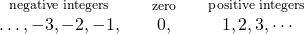### Fractions

Often in life, whole amounts are not exactly what we need. A baker must use a little more than a cup of milk or part of a teaspoon of sugar. Similarly a carpenter might need less than a foot of wood and a painter might use part of a gallon of paint. These people need to use numbers which are part of a whole. These numbers are very useful both in algebra and in everyday life and they are called factions. Hence, Fractions are a way to represent parts of a whole. It is written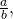whereandare integers andIn a fraction,is called the numerator andis called the denominator. The denominatorrepresents the number of equal parts the whole has been divided into, and the numeratorrepresents how many parts are included. The denominator,cannot equal zero because division by zero is undefined.

In (Figure), the circle has been divided into three parts of equal size. Each part representsof the circle. This type of model is called a fraction circle. Other shapes, such as rectangles, can also be used to model fractions.Doing the Manipulative Mathematics activity Model Fractions will help you develop a better understanding of fractions, their numerators and denominators.

What does the fractionrepresent? The fractionmeans two of three equal parts.An interactive or media element has been excluded from this version of the text. You can view it online here: http://pressbooks.oer.hawaii.edu/buildingmaint/?p=142

#### Improper and Proper Fractions

In an improper fraction, the numerator is greater than or equal to the denominator, so its value is greater than or equal to one. Fractions such as,,, andare called improper fractions.

When a fraction has a numerator that is smaller than the denominator, it is called a proper fraction, and its value is less than one. Fractions such as,, andare proper fractions.

#### Equivalent Fractions

Equivalent fractions are fractions that have the same value. For example, the fractionsandhave the same value, 1. Figure shows two images: a single pizza on the left, cut into two equal pieces, and a second pizza of the same size, cut into eight pieces on the right. This is a way to show thatis equivalent to. In other words, they are equivalent fractions.Figure #. Since the same amount is of each pizza is shaded, we see thatis equivalent to.

To add or subtract fractions, they must have a common denominator. If the factions have the same denominator, we just add the numerators and place the sum over the common denominator. If the fractions have different denominators, what do we need to do?

First, we will use fraction tiles to model finding the common denominator ofandWe’ll start with onetile andtile. We want to find a common fraction tile that we can use to match bothandexactly.

If we try thepieces,of them exactly match thepiece, but they do not exactly match thepiece.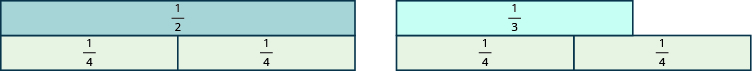If we try thepieces, they do not exactly cover thepiece or thepiece.If we try thepieces, we see that exactlyof them cover thepiece, and exactlyof them cover thepiece.If we were to try thepieces, they would also work.Even smaller tiles, such asandwould also exactly cover thepiece and thepiece.

The denominator of the largest piece that covers both fractions is the least common denominator (LCD) of the two fractions. So, the least common denominator ofandis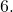Notice that all of the tiles that coverandhave something in common: Their denominators are common multiples ofandthe denominators ofandThe least common multiple (LCM) of the denominators isand so we say thatis the least common denominator (LCD) of the fractionsand. Therefore, the least common denominator (LCD) of two fractions is the least common multiple (LCM) of their denominators.

To find the LCD of two fractions, we will find the LCM of their denominators. We follow the procedure we used earlier to find the LCM of two numbers. We only use the denominators of the fractions, not the numerators, when finding the LCD.

Find the LCD for the fractionsandSolution
 Factor each denominator into its primes.List the primes of 12 and the primes of 18 lining them up in columns when possible.Bring down the columns.Multiply the factors. The product is the LCM.The LCM of 12 and 18 is 36, so the LCD ofand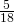is 36. LCD ofandis 36.

3.1: Required Math Concepts is shared under a CC BY 4.0 license and was authored, remixed, and/or curated by Clifford Rutherford via source content that was edited to conform to the style and standards of the LibreTexts platform; a detailed edit history is available upon request.